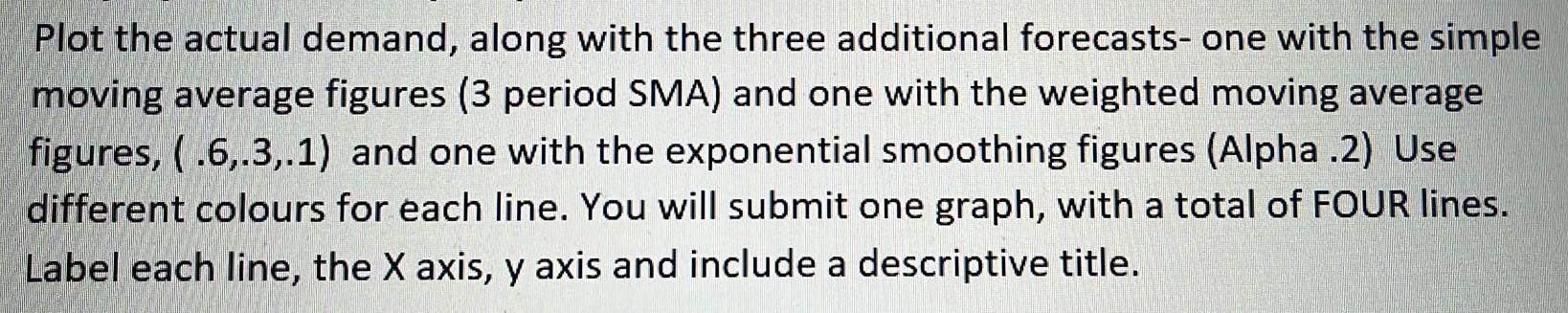# (Solved): Plot the actual demand, along with the three additional forecasts- one with the simple moving a ...Plot the actual demand, along with the three additional forecasts- one with the simple moving average figures ( 3 period SMA) and one with the weighted moving average figures, and one with the exponential smoothing figures (Alpha .2) Use different colours for each line. You will submit one graph, with a total of FOUR lines. Label each line, the axis, axis and include a descriptive title.

We have an Answer from Expert